## Quiz 3 : Preparing Your Taxes Part IILooking for Finance Homework Help?# Quiz 3 : Preparing Your Taxes Part II

The following table A1 shows the information about the income of Mrs. MW. The taxable income, tax liability and tax refund or tax owed is calculated as follows: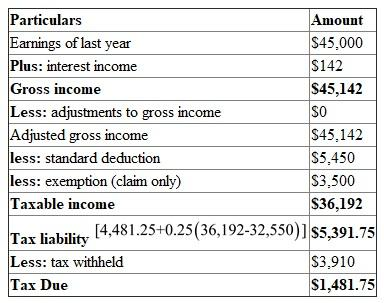In table A1 , the gross income is obtained by adding the earnings of last year and interest incomes. In table A1 , the taxable income is obtained by subtracting gross income from the standard deviation and exemptions. In table A1, the tax liability is obtained as follows: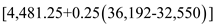Therefore, the tax liability isIn table A1 , the tax due is calculated by subtracting tax liability form the tax withdrawals Therefore, from the above calculations it can be concluded that, the taxable income is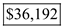tax liability is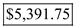and tax refund or tax owed isa) The following table S1 shows the calculations of gross income of Mrs. DF which is as follows: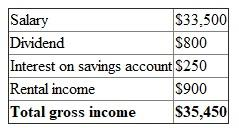In table S1, the gross income is obtained by adding the salary, dividend, interest on savings account, and rental income Hence, the gross income is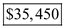b) The following table S2 shows the calculation of the total tax exempted income, which is as follows: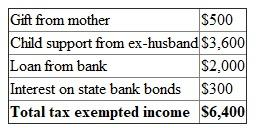In the table S2 the t otal tax exempted income is calculated by adding all the particulars i.e. gift from mother, child support from ex-husband, loan from bank, and interest on state bank bonds Hence, the total tax exempted inco me is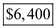a) The following table D1 shows the calculation of capital gains , which is as follows: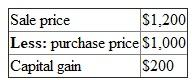In table D1 , the capital gain is calculated by subtracting sales price from purchases price Now calculate tax due by multiplying capital gains to 28 percent tax, which is as follows: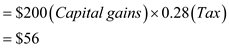Therefore, from the above calculations it can be concluded that, Mrs. DF need to pay tax due of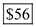The stock was held for less than one year, i:e 5 months, therefore, the gain is a short-term capital gain and taxed at ordinary income tax rates. b) The following table D2 shows the calculation of capital gains , which is as follows: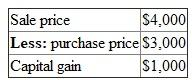In the table D2 , the capital gains are calculated by subtracting sales price from purchases price Now calculate tax due by multiplying the capital gain form 15 percent tax, which is as follows: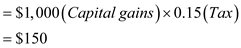The bond was held for more than one year, and less than 5 year, hence, the gain is a long-term capital gain and taxed at 15 percent Therefore, from the above calculations it can be concluded that Mrs. DF need to pay tax due of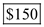c) The following table D3 shows the calculation of capital gains , which is as follows: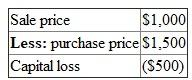In the table D3 , the capital loss is calculated by subtracting sales price from purchases price Now, as there is a capital loss calculate Tax savings by multiplying the capital gain form 28 percent tax which is as follows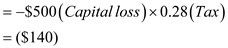Therefore, from the above calculations it can be concluded that, there is a capital loss of \$500. At 28 percent, Mrs. DF would pay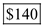less in taxes.